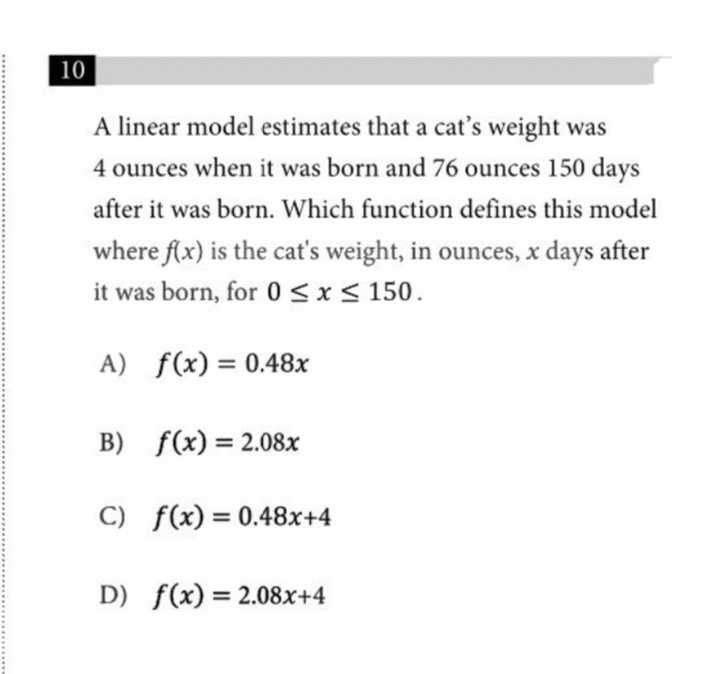### ¿Todavía tienes preguntas de matemáticas?

Pregunte a nuestros tutores expertos
Algebra
PreguntaA linear model estimates that a cat's weight was $$4$$ ounces when it was born and $$76$$ ounces $$150$$ days after it was born. Which function defines this model where $$f ( x )$$ is the cat's weight, in ounces, $$x$$ days after it was born, for $$0 \leq x \leq 150$$

A) $$f ( x ) = 0.48 x$$

B) $$f ( x ) = 2.08 x$$

C) $$f ( x ) = 0.48 x + 4$$

D) $$f ( x ) = 2.08 x + 4$$